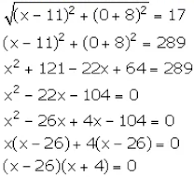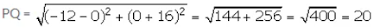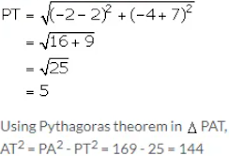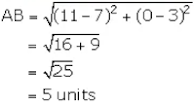# ICSE Solutions for Selina Concise Chapter 28 Distance Formula Class 9 Maths

### Exercise 28

1 .Find the distance between the following pairs of points:

(i) (-3, 6) and (2, -6 )
(ii) (-a, -b) and (a, b)
(iii) (3/5 ,2) and (-1/5 , 1 ²/5)
(iv) (√3+1, 1) and (0, √3)

(i) (-3 , 6) and (2, -6)
Distance between the given points

(ii) (-a, -b) and (a, b)
Distance between the given points

(iv) (√3 + 1, 1) and (0, √3)
Distance between the given points

2. Find the distance between the origin and the point:

(i) (-8, 6)
(ii) (-5, -12)
(iii) (8, -15)

3 . The distance between the points (3, 1) and (0, x) is 5. Find x.

It is given that the distance between the points A(3, 1) and B(0, x) is 5.
∴ AB = 5

AB2 = 25

⇒ (0-3)2 + (x-1)2 = 25

⇒ 9 + x2 + 1 – 2x = 25

⇒ x2 – 2x – 15 = 15

⇒ x2 – 5x + 3x – 15 = 0

⇒ x(x-5) + 3(x-5) = 0

⇒ (x-5) (x+3) = 0

⇒ x = 5, -3

4. Find the co-ordinates of points on the x-axis which are at a distance of 17 units from the point (11, -8).

Let the coordinates of the point on x - axis be (x,0).
From the given information, we have :⇒ x = 26 , -4
Thus, the required co - ordinates of the points on x -axis are (26, 0) and (-4, 0).

5 . Find the coordinates of the points on the y-axis, which are at a distance of 10 units from the point (-8, 4).

Let the coordinates of the point on y -axis be (0, y).
From the given information, we have :⇒ y = 10, -2
Thus, the required co - ordinates of the points on y -axis are (0, 10) and (0, -2).

6. A point A is at a distance of √10 unit from the point (4, 3). Find the co-ordinates of point A, if its ordinate is twice its abscissa.

It is given that the co - ordinates of point A are such that its ordinate is twice its abscissa.
So, let the co - ordinates of point A be (x , 2x).
We have :⇒ x = 1, 3
Thus, the co -ordinates of the point A are (1, 2) and (3, 6).

7. A point P (2, -1) is equidistant from the points (a, 7) and (-3, a). Find a.

Given that the point P (2, -1) is equidistant from the points A (a, 7) and B(-3, a).
∴ PA = PB

PA2 = PB2

⇒ (a-2)2 + (7+1)2 = (-3-2)2 + (a+1)2

⇒ a2 + 4 – 4a + 64 = 25 + a2 + 1 + 2a

⇒ 42 = 6a
⇒ a = 7

8. What point on the x-axis is equidistant from the points (7, 6) and (-3, 4)?

Let the co- ordinates of the required point on x - axis be P (x, 0).
The given points are A(7, 6) and B(-3, 4).
Given, PA = PB

PA2 = PB2

⇒ (x-7)2 + (0-6)2 = (x+3)2 + (0-4)2

⇒ x2 + 49 – 14x + 36 = x2 + 9 + 6x + 16

⇒ 60 = 20 x
⇒ x = 3
Thus, the required point is (3, 0).

9. Find a point on the y-axis which is equidistant from the points (5, 2) and (-4, 3).

Let the co - ordinates of the required point on y - axis be P(0,y).
The given points are A(5, 2) and B(-4, 3).
Given, PA = PB

PA2 = PB2

⇒ (0-5)2 + (y-2)2 = (0+4)2 + (y-3)2

⇒ 25 + y2 + 4 – 4y = 16 + y2 + 9 – 6y

⇒ 2y = -4
⇒ y = -2
Thus, the required point is (0, -2).

10. A point P lies on the x-axis and another point Q lies on the y-axis.

(i) Write the ordinate of point P.
(ii) Write the abscissa of point Q.
(iii) If the abscissa of point P is -12 and the ordinate of point Q is -16; calculate the length of line segment PQ.

(i) Since, the point P lies on the x - axis, its ordinate is  0.
(ii) Since, the point Q lies on the y - axis, its abscissa is 0.
(iii) The co - ordinates of p and Q are (-12, 0) and (0, -16) respectively.11. Show that the points P (0, 5), Q (5, 10) and R (6, 3) are the vertices of an isosceles triangle.

12. Prove that the points P (0, -4), Q (6, 2), R (3, 5) and S (-3, -1) are the vertices of a rectangle PQRS

13. Prove that the points A (1, -3), B (-3, 0) and C (4, 1) are the vertices of an isosceles right-angled triangle. Find the area of the triangle.Hence, A, B, C are the vertices of a right - angled triangle.
Hence, △ABCD is an isosceles right - angled triangle.
Area of △ABC = (1/2) × AB ×CA
= (1/2) × 5×5
= 12.5 sq. units

14. Show that the points A (5, 6), B (1, 5), C (2, 1) and D (6, 2) are the vertices of a square ABCD.

15. Show that (-3, 2), (-5, -5), (2, -3) and (4, 4) are the vertices of a rhombus.

16. Points A (-3, -2), B (-6, a), C (-3, -4) and D (0, -1) are the vertices of quadrilateral ABCD; find a if ‘a’ is negative and AB = CD.

AB = CD

⇒ AB2 = CD2

⇒ (-6+3)2 + (a+2)2 = (0+3)2 + (-1+4)2

⇒ 9 + a2 + 4 + 4a = 9+9

⇒ a2 + 4a – 5 = 0

⇒ a2 - a + 5a - 5 = 0

⇒ a(a-1) + 5(a - 1) = 0
⇒ (a - 1)(a+5) = 0
⇒ a = 1 or -5
It is given that a is negative, thus the value of a is -5.

17. The vertices of a triangle are (5, 1), (11, 1) and (11, 9). Find the co-ordinates of the circumcentre of the triangle.

Let the circumcntre be P (x, y).
Then, PA = PB

⇒ PA2 = PB2

⇒ (x-5)2 + (y-1)2 = (x-11)2 + (y-1)2

⇒ x2 + 25 – 10x = x2 + 121 – 22x

⇒ 12x = 96

⇒ x = 8

Also, PA =PC
⇒ PA2 = PC2

⇒ (x-5)2 + (y-1)2 = (x-11)2 + (y-9)2

⇒ x2 + 25 – 10x + y2 + 1 – 2y = x2 + 121 – 22x + y2 + 81 – 18y

⇒ 12 x + 16 y = 176
⇒ 3x + 4y = 44
⇒ 24 + 4y = 44
⇒ 4y = 20
⇒ y = 5
Thus, the co - ordinates of the circumcentre of the triangle are (8, 5).

18. Given A = (3, 1) and B = (0, y – 1). Find y if AB = 5.

AB = 5

⇒ AB2 = 25

⇒ (0-3)2 + (y-1-1)2 = 25

⇒ 9 + y2 + 4 – 4y = 25

⇒ y2 -4y – 12 = 0

⇒ y2 – 6y + 6y - 12 = 0

⇒ y(y-6) + 2(y - 6) = 0
⇒ (y - 6)(y +2) = 0
⇒ y = 6, -2

19. Given A = (x + 2, -2) and B (11, 6). Find x if AB = 17.

AB = 17

⇒ AB2 = 289

⇒ (11-x-2)2 + (6+2)2 = 289

⇒ x2 + 81 – 18x + 64 = 289

⇒ x2 -18x – 144 = 0

⇒ x2 – 24x + 6x - 144 = 0

⇒ x(x - 24) + 6(x - 24) = 0
⇒ (x -24)(x + 6) = 0
⇒ x = 24, -6

20. The centre of a circle is (2x – 1, 3x + 1). Find x if the circle passes through (-3, -1) and the length of its diameter is 20 unit.

Distance between the points A (2x -1, 3x + 1) and B(-3 , -1) = Radius of circle
∴AB = 10(Since, diameter  = 20 units, given)

21. The length of line PQ is 10 units and the co-ordinates of P are (2, -3); calculate the co-ordinates of point Q, if its abscissa is 10.

Let the co - ordinates of point Q be (10, y).
PQ = 10

PQ = 10

⇒ PQ2 = 100

⇒ (10-2)2 + (y+3)2 = 100

⇒ 64 + y2 + 9 + 6y = 100

⇒ y2 + 6y – 27 = 0

⇒ y2 + 9y – 3y - 27 = 0

⇒ y(y +9) -3(y + 9) = 0
⇒ (y + 9)(y -3) = 0
⇒ y = -9, 3
Thus, the required co- ordinates of point Q are (10, - 9) and (10, 3).

22. Point P (2, -7) is the center of a circle with radius 13 unit, PT is perpendicular to chord AB and T = (-2, -4); calculate the length of:

(i) AT

(ii) AB

(i) Given, radius = 13 units
∴ PA = PB = 13 units
Using distance formula,AT = 12 units

(ii) We know that the perpendicular from the centre of a circle to a chord bisects the chord.
∴ AB = 2AT = 2×12 units = 24 units

23. Calculate the distance between the points P (2, 2) and Q (5, 4) correct to three significant figures.

24. Calculate the distance between A (7, 3) and B on the x-axis whose abscissa is 11.

We know that any point on x -axis has coordinates of the form (x, 0).
Abscissa of point B = 11
Since, B lies of x - axis, so its co -ordinates are (11, 0).25. Calculate the distance between A (5, -3) and B on the y-axis whose ordinate is 9.

We know that any point on y - axis has coordinates of the form (0 ,y).
Ordinate of point B = 9
Since, B lies of y - axis, so its co - ordinates are (0, 9).

26. Find the point on y-axis whose distances from the points A (6, 7) and B (4, -3) are in the ratio 1: 2.

Let the required point of y - axis be P(0

Thus, the required points on  y - axis are (0, 9) and (0, 35/3).

27. The distances of point P (x, y) from the points A (1, –3) and B (-2, 2) are in the ratio 2: 3.
Show that: 5x2 + 5y2 – 34x + 70y + 58 = 0.